• Call Now

1800-102-2727•

# Stefan Boltzmann law, explanation, mathematical formulation, solved examples, FAQs

Ever wondered how we can measure the temperature of distant stars like the Sun which have temperatures high enough that you can’t use a thermometer or pyrometer of any kind. Since the Sun emits radiation all the time perhaps there can be a way to use those to calculate the temperature. This can be done by having the knowledge about Stefan Boltzmann law.

• Stefan Boltzmann law
• Rate of cooling according to Stefan–Boltzmann law
• Temperature of the Sun and Solar Constant
• Practice problem
• FAQs

## Stefan Boltzmann law

The radiant energy emitted by a perfectly black body per unit area per time is directly proportional to the fourth power of its absolute temperature.

The energy emitted by a black body is given by,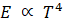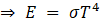Where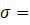Stefan’s constant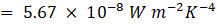Dimensions for Stefan’s constant is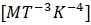.

For an ordinary body,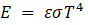Where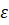is the emissivity of the material.

If Q is the total radiant energy radiated by the body, then we get,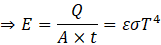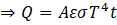The radiant power is defined as the radiant energy per unit time.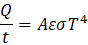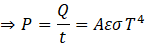For a body at temperature T and its surrounding temperature To, the body does absorption and emission of energy simultaneously.

The energy emitted by the body is given as follows: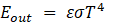The energy absorbed by the body is given as follows: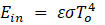The net energy emitted if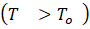is given as follows: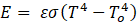## Rate of cooling according to Stefan–Boltzmann law

Net rate of radiation energy loss (for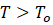),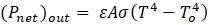This energy loss results in the decrement of the temperature of the body of specific heat capacity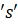as follows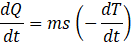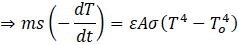Rate of cooling: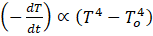## Temperature of the Sun and Solar Constant

Let R be the radius of the Sun and T be its temperature.

Area of the Sun,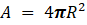The energy emitted per second through the radiations is given by,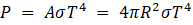On reaching the Earth, this energy (P) will spread over a sphere around the Sun of radius r, where r is the average distance between the Earth and the Sun.

Solar constant (S)

The intensity of the solar radiation at the surface of the Earth is known as the solar constant.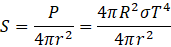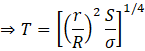By substituting the values, we get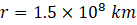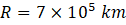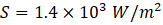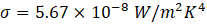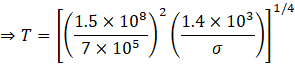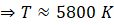## Practice problems

Q.

A body of emissivity 0.75, surface area 300 cm2, and temperature 227 is kept in a room at temperature 27. Calculate the initial value of the net power emitted by the body.

1. 50 W
2. 59.4 W
3. 69.4 W
4. 79.4 W

A.

Given,

Emissivity of the body, ε = 0.75

Surface area of the body, = 300 cm2 = 0.03 m2

Temperature of the body, = 227℃ = 227 + 273 = 500 K

Temperature of the surrounding, T0= 27℃ = 27 + 273 = 300 K

The net radiant power of the body is given as follows:

Pnet = ε𝜎A(4 - T04)

Pnet = 0.75 × 5.67 × 10-8 × 0.03× ( 5004 - 3004)

Pnet = 69.4 W

Q. A spherical ball A of surface area 20 cm2 is kept at the centre of a hollow spherical shell B of area 80 cm2. The surface of A and the inner surface of B emit as black bodies. Both A and B are at 300 K

(a) How much is the radiation energy emitted per second by ball A?

(b) How much is the radiation energy emitted per second by the inner surface of B?A.

Both spherical bodies A and B are maintained at 300 K and the surface area of B is four times as much as the surface area of A.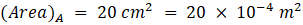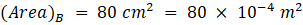The black bodies in consideration are: (i) the outer surface of body A, (ii) the inner surface of body B.

(a)

According to Stefan’s law,

Rate of emission of the radiation energy by body A,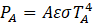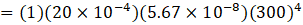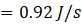or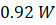The radiation energy emitted by body A per second is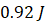.

(b)

According to Stefan’s law,

Rate of emission of the radiation energy by body B,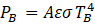Since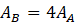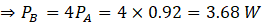The radiation energy emitted by body B per second is 3.68 J.

Q. A copper sphere is suspended in an evacuated chamber maintained at 300 K. The sphere is maintained at a constant temperature of 500 K by heating it electrically. A total of 210 W of electric power is needed to do it. When the surface of the copper sphere is completely blackened, 700 W is needed to maintain the same temperature of the sphere. Calculate the emissivity of copper.A.

Power required to maintain the temperature of copper sphere,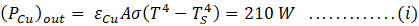Power required to maintain the temperature of sphere after blackening of its surface,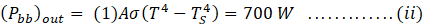Dividing equation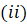by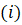, we get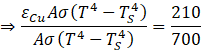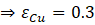Q. The power radiated by a black body is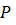and it radiates maximum energy at wavelength,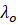. If the temperature of the black body is now changed so that it radiates the maximum energy at wavelength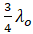, the power radiated by it becomes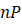. Find the value of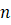1.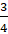2.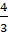3.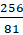4.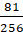A.

According to Wien’s displacement law,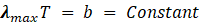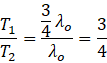Power radiated by a blackbody,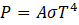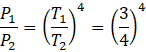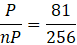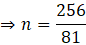Thus, option (C) is the correct answer.

## FAQs

Q. A hot metallic sphere of radius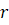radiates heat. Does the rate of cooling depend upon radius?

A. Yes

Rate of cooling,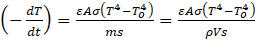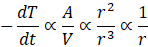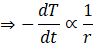Q. A sphere, a cube and a thin circular plate are made of the same material and all have the same mass. These are heated to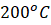and then placed in a room. Then the temperature of which object out of three will drop to room temperature at last?
A.  Since the mass of all three objects is the same, therefore the rate of cooling will be proportional to their surface area. Since for a given volume the surface area of the sphere is least, the rate of cooling will be the least for the sphere. Hence the temperature of the sphere will drop to room temperature at last.

Q. What is the dimensional formula for Stefan’s constant?
A.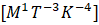Q. What are gray bodies?
A. Real objects that radiate less heat as compared to a perfect black body are known as gray bodies. Emissivity for such objects ranges from 0 to 1.Talk to our expert
Resend OTP Timer =
By submitting up, I agree to receive all the Whatsapp communication on my registered number and Aakash terms and conditions and privacy policy